#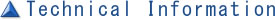##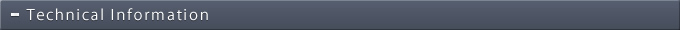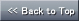### Chiller

Series Top

#### Piping pressure sample calculation

Example 1 In the example on the right, claiming for plumbing loss.
1. First, determine the equivalent length of straight plumbing pipe.
1. Determine the full length of the pipe.
(Full length)=3+4+2+10+5+6+2=32(m) since going and coming back is there
2. Equivalent straight length of the tube joint is calculated using the chart.
Screw type 90°Since short elbow`s 25 is1.6m(joint)=1.6×6=9.6(m)
3.1, 2 total is calculated.
64+9.6=73.6(m)
2. Next, from flow rate and pipe diameter, hydraulic loss is determined.
From the graph, the intersection of 25A line and 30［ℓ/min］ is 0.04
Multiply 3.1 and 2
73.6×0.04=2.944≒3(m) ※ In case of old steel pipe, it is 1.2～2.5 times.
4. From Chiller to equipment, determine rise in the height.
4+10=14(m)
Find the total of 5.3 and 4.
3+14=17(m)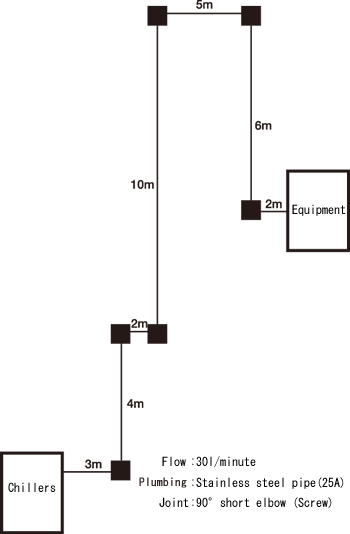Example 2 In the example on the right, claiming for plumbing grit loss.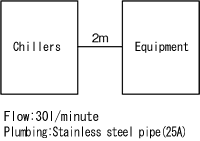1. 8A

2. 15A
3. 20A
4. 32A

1. First, determine the equivalent length of straight plumbing pipe. (Full length) = 2(m)
2. Next, from flow rate and pipe diameter, hydraulic loss is determined.
From the graph, the intersection is 30(ℓ/min) and each piping diameter is
1. at 8A 8.4
2. at 15A 0.55
3. at 20A  0.13
4. at 32A  0.01
3. 1 and 2 multiply. *In case of old steel pipe etc., it is 1.2～2.5 times.
1. at 8A  2×8.4=16.8(m)
2. at 15A 2×0.55=1.1(m)
3. at 20A 2×0.13=0.26(m)
4. at 32A 2×0.01=0.02(m)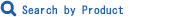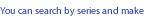Defined Search